# Physics - Sound Part II

## Introduction

• Sound is a form of energy, which produces a sensation when we hear it in our ears.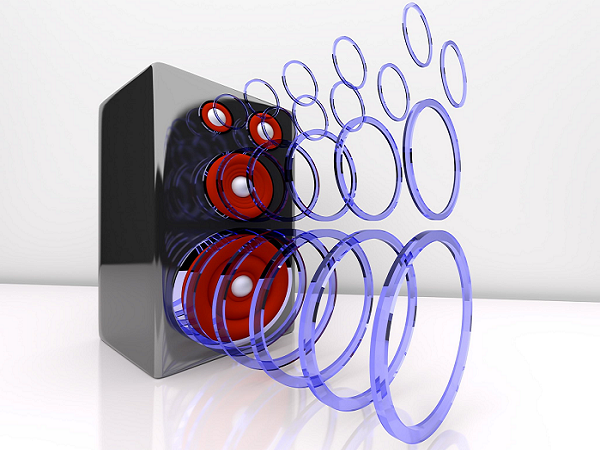• Sound can be produce in number of ways, such as, by plucking, scratching, rubbing, blowing or shaking different objects.

• The sound of the human voice is produced because of the vibrations in the vocal cords.

• Sound waves are typically characterized by the motion/vibration of particles in the medium and hence known as mechanical waves.

• Sound waves oscillate back and forth on their position; hence, they are known as longitudinal waves.

## Propagation of Sound

• The substance or object through which sound is transmitted is known as medium.

• Sound moves through a medium from the point of the generation to the listener; the sound medium could be solid, liquid, or gas.

• However, sound cannot travel through a vacuum medium.

• The particles (of gas, liquid, or solid) do not travel all the way from the vibrating object to the ear, but rather when the object vibrates, it sets the particles of the medium around it vibrating and so on and so forth.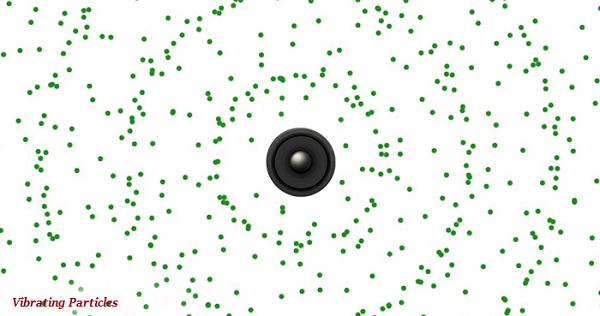• In other words, the particles of the medium do not travel/move forward, but rather the disturbance is carried forward through one vibrating particle to another.

• When vibrating particles move forward, they push and compress the air in front of it and create a region of high pressure known as compression (see the image given below).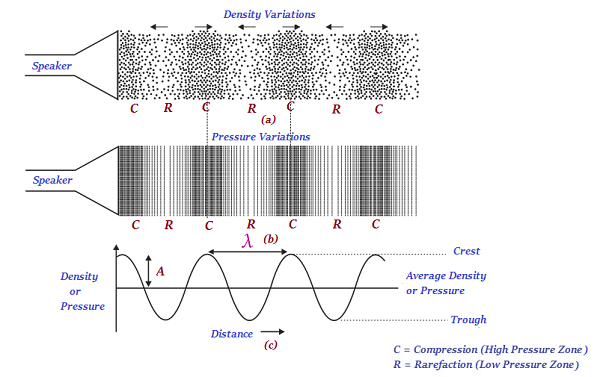• Further, when the vibrating particles move backwards, it creates a region of low pressure known as rarefaction (R) (see the image given above).

• As the particles move back and forth rapidly, a series of compressions (high pressure zone) and rarefactions (low pressure zone) is created in the air; likewise, the sound wave propagates through the medium.

• As shown in the image given above, the lower portion (valley) of the curve is known as trough and the upper portion (peak) is known as crest.

• The distance between two consecutive compressions or two consecutive rarefactions is known as the wavelength.

• Wavelength is usually represented by the Greek letter lambda (λ) and its SI unit is meter (m).

• The number of the compressions or rarefactions that counted per unit time is known as frequency of the sound wave.

• Frequency of the sound wave is commonly represented by ν (Greek letter, nu).

• The SI unit of frequency of the sound wave is hertz (Hz).

• The sensation of a frequency that we sense/listen is usually referred as the pitch of a sound.

• The faster the vibration of the sound source, the higher is the frequency and so the higher is the pitch (see the image given below).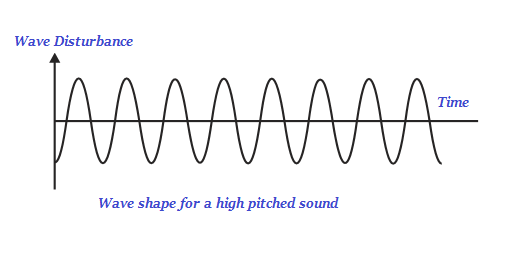• Likewise, a high pitch sound has more number of compressions and rarefactions passing the fixed point per unit time.

• The lower the vibration of the sound source, the lesser is the frequency and so the lesser is the pitch (see the image given below).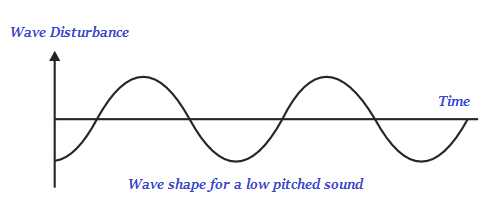• Likewise, a lower pitch sound has less number of compressions and rarefactions passing the fixed point per unit time.

• The magnitude of the maximum disturbance in the given medium on either side of the mean value is known as the amplitude of the sound wave.

• Amplitude is commonly represented by the letter A.

• The softness or loudness of a sound is fundamentally determined by its amplitude.

• A sound of single frequency is known as tone.

• The sound, which is created by mixing of several harmonious frequencies, is known as note.

• Note is pleasant in listening.